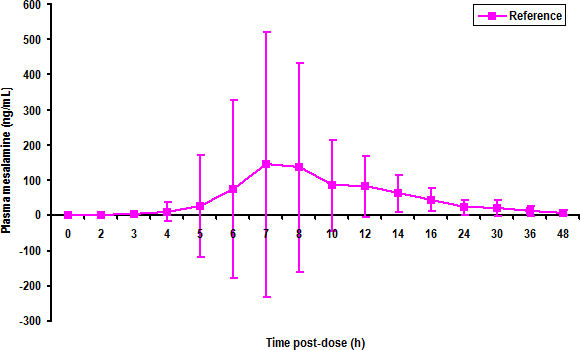## Concentration Statistics - BQL substitution [General Sta­tis­tics]

Hi Sara,

❝ What is your opinion on below quantification limit (BQL) substitution for concentration data summary statistics, by timepoint?

You can use the median and quartiles or $$\small{\bar{x}_{geo}\mp SD_{geo}}$$ if a certain percentage of samples are measurable (I have seen SOPs with 50%, 67%, and 75%) and nothing (‘not reportable’) otherwise. At the end of the day it’s not important at all (not relevant for the BE assessment). Use whatever you like. 1

❝ Zero substitution is the one I have seen the most, …

To quote Harold Boxenbaum (Crystal City workshop about bioanalytical method validation, Arlington 1990):

After a dose we know only one thing for sure: The concentration is not zero.

Ended in shouting matches.❝ … I don't think it is suitable for the calculation of geometric means.

Correct, since$$\lim_{x \to 0} \log x=-\infty.$$For simplicity we can say that $$\small{\log 0}$$ is undefined. It is reasonable to assume that concentrations ($$\small{x \in \mathbb{R}^+}$$) follow a lognormal distribution, and the geometric mean would be the best estimator of location. Some people chicken out, set BQLs to zero, and present arithmetic means. This leads to funny plots with $$\small{\bar{x}\mp SD,}$$ where the lower whisker reaches far below zero. 2 Phew, negative concentrations? Not in this universe.

1. Phoenix/WinNonlin by default calculates descriptive statistics only for numeric values. This can lead to strange results. Say, we have $$n-2$$ values which are BQL and two $$\small{C\geq LLOQ}$$. Then we end up with $$\small{\bar{C}_{ar}=\tilde{C}=\tfrac{C_1+C_2}{2},\bar{C}_{geo}=\sqrt{C_1\times C_2}}$$. Doesn’t make sense. However, we can specify different rule sets for descriptive statistics and plots.
2. A goody from the FDA’s NDA 204-412 (mesalamine delayed release capsules, n = 238, sampling times: pre-dose, 2, 3, 4, 5, 6, 7, 8, 10, 12, 14, 16, 24, 30, 36, and 48 h post-dose). BQLs were imputed as LLOQ/2.Splendid, $$\small{\bar{x}\mp SD}$$ in bloody Excel. Hey, wait a minute, that’s a fucking line plot… Oh dear! One hour intervals in the beginning are as wide as the 12 hours at the end.

Let’s see the XY-plot:Do these guys and dolls really believe that at seven hours there’s a ~16% chance that concentrations are 232 and a ~1% chance that concentrations are 731‽ Any statistic implies an underlying distribution. The arithmetic mean implies a normal distribution with $$\small{x \in \mathbb{R}\:\vee\:x \in \left \{-\infty, +\infty\right \}}$$. Fantastic.

Which cult of Pastafarianism do they belong to?
The one holding that negative mass exist or the one believing in negative lengths?$$\small{\bar{x}_{geo}\mp SD_{geo}}$$ reflects the terrible variability of this drug much better and shows that high concentrations are more likely than low ones.

Dif-tor heh smusma 🖖🏼 Довге життя Україна!Helmut SchützThe quality of responses received is directly proportional to the quality of the question asked. 🚮
Science QuotesIng. Helmut Schütz